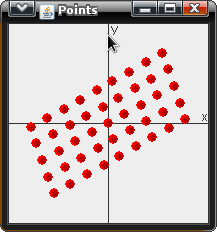3D Rotation Matrix - Graph 3D

math

This was a simple program I wrote that Displays 3D points. It does so by simply projecting a 3D (vector) into 2D. The Jar file can be downloaded here: Graph3D.jar The C/C++ version can be found hereBefore looking at the source, let's take a look at some of the fundamental mathematics behind the software. rotations - Rotations in this software simple geometric transformations based around an unmoving center axis. Below are the three rotation matrices for each axis, X, Y, and Z, respectively. Every operation can be found in Transform.java below.

Rotate Around X,Y, or Z Axis

$$rotX = \left[\begin{matrix} 1 & 0 & 0 \\ 0 & cos(\theta) & -sin(\theta) \\ 0 & sin(\theta) & cos(\theta) \\ \end{matrix}\right]$$

$$rotY = \left[\begin{matrix} cos(\theta) & 0 & sin(\theta) \\ 0 & 1 & 0 \\ -sin(\theta) & 0 & cos(\theta) \\ \end{matrix}\right]$$

$$rotZ = \left[\begin{matrix} cos(\theta) & -sin(\theta) & 0 \\ sin(\theta) & cos(\theta) & 0 \\ 0 & 0 & 1 \\ \end{matrix}\right]$$

It is also important to know that every point in our world is defined by:

$$\vec{p} = \left[\begin{array}{c} x \\ y \\ z \\ \end{array}\right]$$

This can be seen in Point3D.java below. (Which is synonymous to a Vector in this application.)

Rotate Around Arbitrary Axis

The above rotations are all rotations about either the X,Y, or Z axises. But another common and more complicated rotation is to rotate Vector/Point A around Vector/Point B.

Here are the Steps:

1. the Vector that is being rotated around must be NORMALIZED. This can be done very easily

$$d = \sqrt{x^2 + y^2 + z^2}$$

$$\hat{p} = \left[\begin{array}{c} x/d \\ y/d \\ z/d \\ \end{array}\right]$$

1. next, using quaternions, perform the rotation. Where:

$$\hat{p} = ai + bj + ck$$

$$q0 = cos(\theta/2), q1 = sin(\theta/2) a, q2 = sin(\theta/2) b, q3 = sin(\theta/2) c, (\theta\ is\ in\ radians)$$

The rotation matrix evaluates to the following:

$$rotAB = \left[\begin{array}{ccc} (q0^2 + q1^2 - q2^2 - q3^2) & 2(q1q2 - q0q3) & 2(q1q3 + q0q2) \\ 2(q2q1 + q0q3) & (q0^2 - q1^2 + q2^2 - q3^2) & 2(q2q3 - q0q1) \\ 2(q3q1 - q0q2) & 2(q3q2 + q0q1) & (q0^2 - q1^2 - q2^2 + q3^2) \\ \end{array}\right]$$

Example:

Suppose we have point:

$$\vec{p} = \left[\begin{array}{c} 1 \\ 2 \\ 3 \\ \end{array}\right]$$

and we would like to rotate $$\vec{p}$$ by 30° around the X axis.

$$\vec{p}_{new} = (rotX)\vec{p} = \left[\begin{array}{ccc} 1 & 0 & 0 \\ 0 & cos(\theta) & -sin(\theta) \\ 0 & sin(\theta) & cos(\theta) \\ \end{array}\right] \left[\begin{array}{c} x \\ y \\ z \\ \end{array}\right] = \left[\begin{array}{ccc} 1 & 0 & 0 \\ 0 & cos(30) & -sin(30) \\ 0 & sin(30) & cos(30) \\ \end{array}\right] \left[\begin{array}{c} 1 \\ 2 \\ 3 \\ \end{array}\right]$$

And finally, to project the 3D points onto a 2D canvas after performing a rotation, a simple way is to simply ignore the Z coordinate and draw the point based on it's X and Y coordinates. However this is assuming that you're projecting it on to the screen as if you are looking straight at it.

The source code can be found below as well as being bundled into the Jar file.

Cube 3D - A simple 3D Cube engine that demonstrates the usage of ALL the above mentioned equations.

Transform3D.java - This is a simple version using a 3x3 matrix. Using a 4x4 matrix you can also store the translation information. This is useful when trying to program a skeleton represented by vectors. (Also, check out Graph/Rotate4D here

Transform.java

Point3D.java

Other included source:

Graph3D.java